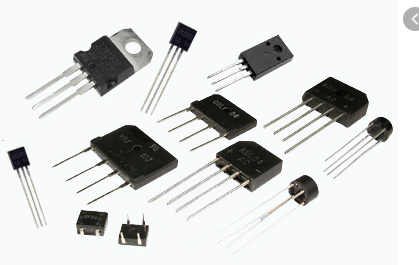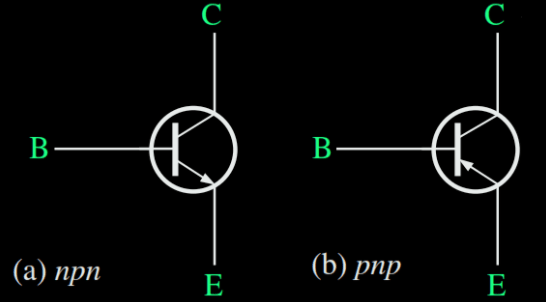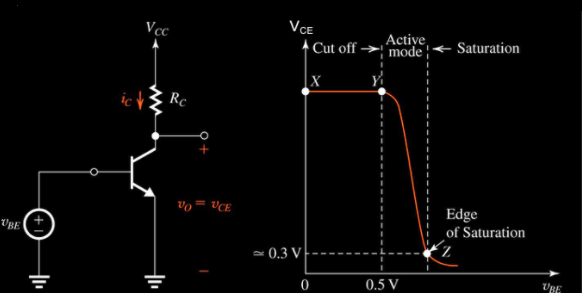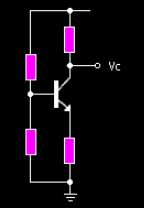# NPN Transistor Working and Application Explained

NPN transistor is a three-terminal device having a p-type semiconductor sandwiched between the two n-type semiconductors. It is the most useful of the two bipolar junction devices. The other being the PNP transistor. It has various applications and is used mostly for amplification and switching.

Well, before moving into the concept of NPN transistors, let us have a little knowledge about the basics of transistors.

## Transistors

Basically, a transistor is an electronic device helpful in amplification and electronic switching. It is a three-terminal device. When we apply a current or voltage at one pair of the terminals, the other terminal pair current controls it.Transistors

As we are saying that it works as an amplifier, so I hope you can predict if the value at output would be higher or lower than the input.

Yes, it would be higher.

The different types of transistors are classified based on structure, electrical polarity, maximum power rating, etc. Depending on the structure, it could be MOSFET, BJT, JFET, IGBT, and others.

But in this particular article, our focus is the bipolar junction transistor, i.e., BJT.

### Bipolar Junction Transistor

BJT is a semiconductor device having three terminals namely, emitter, base, and collector. It is again divided into two parts, NPN and the PNP transistors. Both transistors have a sandwiched layer of one type between the other on both sides.

In NPN, the current direction is from base to emitter and in PNP it is just the opposite, i.e., from the emitter to the base.Bipolar Junction Transistor

Among the two, NPN is the one that is used mostly in the applications. You may ask why?

So the answer is because the mobility of electrons is more than that of the holes.

## NPN Transistor Construction and SymbolSymbol of NPN

Looking at the fig, we can say that an NPN transistor is a combination of two diodes connected together back to back. On the basis of the terminal connection, the two diodes are emitter-base and collector-base diodes respectively. Also, the direction of current in the NPN transistor is from the emitter to the base region.Construction of NPN transistor

The three terminals emitter, base, and collector are all doped differently. The emitter is moderately doped with the least doping in the base region (lightly doped p-semiconductor). The collector region is heavily doped.

Here, the base region has the control for ON/OFF of the transistor based on the input given to it. The emitter region is always connected to the negative supply and the collector with the positive one.

## Working of NPN Transistor

As shown in the fig, the base-emitter junction is made forward biased with a supply voltage as VCC. Also, the collector-base junction is reverse biased and VEE is the supply for this region.

Now we must understand here how to make a junction forward or reverse biased?

As the n-type of semiconductor comes first when talking about the emitter-base region therefore, the negative of the voltage supply gets connected with it to make it in forward bias. Similarly, for the collector-base region, the positive supply of VCC is connected to the n-type semiconductor and thus made reverse biased.

The depletion region of the emitter-base region is smaller as compared to the collector-base region. Please note that the depletion region behaves as the opposition to the flow of current, therefore, the thinner the layer the more the current flow in that region.

In an n-type semiconductor the majority charge carriers are the electrons and thus, start moving towards the base junction, thus the name emitter current, IE.

The base region is very lightly doped and is a p-type semiconductor, therefore has a few holes as the charge carriers. The few electrons coming from the emitter get recombined with the holes but maximum electrons cross the base region and enter the collector region.

The current produced due to the recombination of the electrons and hole is known as the base current, IB. This current is quite small. The current flowing in the collector region because of the remaining electrons flow is the collector current, IC. It has a higher value than the base current.

### Basic Formulae of NPN Transistor

• From the above analysis, we can say that

IE = IB + IC

• The current in an NPN transistor is given by the ratio of collector current to that of the base. This is called DC current gain and represented as,

Therefore, β= IC / IB

• This factor-beta makes the transistor work as an amplifier. Thus this value goes as high as 200 for standard transistors. It is a dimensionless quantity as is the ratio of two currents.
• Alpha is the other factor that is the current gain of the transistor from the collector to the emitter terminal. Therefore, alpha can be defined as:

α= IC / IE

This is also a dimensionless quantity.

So I have a question for you.

What could be the value of alpha? ‘Low’ or ‘High’?

The base is lightly doped and thus the base current is very low. Similarly, with the remaining electrons, the collector current is quite high and the emitter current is the sum of both the currents.

Now, give it a thought.

Yes, it would be near to unity and for a typical low power transistor, this value ranges between 0.95 to 0.99.

## Relationship between α & β

DC current gain, β = IC / IB

Also, IE = IB + IC

And, α = IC / IE

the value of IC from the above equation,

IC = α * IE

IB = IE – (α * IE)

= IE (1- α)

Therefore, β = IC / IB = IC / IE (1 – α)= α / (1 – α)

Similarly, α = β / (β+ 1)

## Numerical Example On NPN Transistor

Now, let’s try to solve these problems and concrete our concepts.

1. A  NPN transistor has a β value of 100. Calculate a) base current b) emitter current c) value of, for switching a resistive load of 40mA.

Here, we have been given the beta value and the collector current.

1. a) β= 100, IC = 40mA

So what’s the formula that connects the given quantity with the one we need to find!

β = IC / IB

Therefore, IB = 40mA / 100 = 400μA

1. b) The emitter current is given by the sum of the two currents, the base, and the collector.

IE = IB + IC = 400 + 40000 = 40400μA.

1. c) α = β / (β+ 1)

Putting the values, = 100/(100+1)= 0.99

Let us see one more example and understand the concept more thoroughly.

2. Suppose an NPN transistor has a base voltage of 15 volts and the input resistor to the base has a value of 90 K Ohm. Calculate the value of the base current of the transistor.

Before solving this question, we must be clear with one thing. The collector voltage must be positive and higher than the emitter voltage. This is because then only the current will flow through the collector-emitter junction.

Also, there will be a voltage drop between the terminals of the collector and the emitter. This drop will be equal to the value of 0.7 volts in the case of a silicon diode (as it is in forward biased mode). Therefore, in order to conduct the transistor, the base voltage must be greater than the 0.7 volts.

Now, coming back to the problem. VBE = 0.7 volts, VB= 15 volts and RB = 90KΩ

IB = ( VB – VBE ) / RB

= (15 – 0.7) / 90K

= 158μA

## Operating Modes of NPN Transistor

Depending on the biasing of the junctions, an NPN transistor has four modes of operation.

• Saturation
• Cut-off region
• Active
• Reverse activeOperating modes of NPN transistor

Since transistors are non-linear devices, therefore the current-voltage curve has distinct characteristics at different points of time. Let us see all these regions in a bit of detail.

### Saturation Region

This happens when the emitter voltage is less than the base voltage and also the collector is less than the base voltage.

VE < VB

VC < VB

Both the diodes are in forward bias in this region and hence the above conditions prevail. Also, this mode is known as the ON mode of the transistor. Therefore, it acts as a short circuit and the current easily flows from the collector to the emitter.

### Cut-off Mode

This case is just the opposite of the previous one. Here the conditions are reversed, i.e., the base voltage is less than both the emitter and the collector current.

VB < VE

VB < VC

This mode refers to the OFF mode of the transistor and therefore, there is no current conduction between the emitter and the collector. Please note that in cut-off mode the transistor behaves as the open circuit.

### Active Mode

This is the most important operating mode of the transistor. Here, only the transistor becomes the amplifier and amplifies the input signal. The voltage condition here is that the base voltage is less than the collector voltage but greater than the emitter voltage.

VC > VB > VE

The more the dc current gain the more is the amplification. We can see this from the below formula:

IC = β * IB

Therefore, if beta has a value of 200 then there will be 200 times amplification of the base current.

Similarly, with the help of the alpha, beta relationship, and the below formula, IE can be calculated:

IC = α * IE

### Reverse Active

Though this is not in much use still having knowledge is a good thing. This is just the opposite of the active region. Therefore the voltage conditions are like:

VE > VB > VC

Here also, the transistor amplifies but the difference is that the direction of current is from emitter to the collector, i.e., in the reverse direction.

## NPN Versus NPN

Well, there are a few things in common. Both are bipolar junction transistors and a current controlled device. Both are used for amplification and circuit switching. The main points are as below:

• PNP stands for Positive Negative Positive. NPN stands for Negative Positive Negative.
• Conduction of NPN is high and that of PNP is low. This is because in NPN conduction takes place with electrons and in PNP it takes place through the holes but obviously the speed of the electron is more & hence the conduction.NPN versus PNP

• In NPN, we supply a positive current to the base, the current flows through the collector to the emitter. Whereas, when a negative current applies at the base terminal of PNP then the current flows from emitter to collector.
• The current direction in NPN is from collector to emitter and in PNP from emitter to collector.
• Switching time is faster in NPN & slower in PNP.
• NPN turns ON when the electron enters the base region. Whereas, PNP transistor turns ON when holes enter the base terminal.
• In both the transistors, the emitter-base junction is forward biased and the collector-base junction is reverse biased.
• The ground signal is high in the case of PNP and low for NPN.
• The emitter arrow is pointed in for the PNP transistor, while it is pointed out in the case of the NPN transistor.

## Applications

• In very high-frequency applications
• Logarithmic converters
• As amplifier
• In Darlington pair circuits
• Temperature sensors
• Used as a Switch

### NPN Transistor as a Switch

This is a common application for NPN transistors. As we have already seen the operating modes of the transistors, therefore the same helps it in becoming a switch. It is either in ON mode when in the saturation region and in OFF mode when in the cut-off region. These devices are used in high power circuits like in motors, etc.

### NPN Transistor as an Amplifier

This is another very common application of this transistor. Here the smaller value at the input is amplified and is taken out from the output of the amplifier. Basically, a smaller voltage value is amplified at the output.

Almost all our mobile phones use transistors for amplification of sound.

### Transistor Matching of BJTTransistor Matching of NPN

This is one more important concept in a transistor. Here, we connect both the NPN and the PNP transistor in a single circuit to get more amplification. This is achieved as NPN conducts in the positive half cycle and the PNP in the negative half cycle of any signal (just like a full-wave bridge rectifier) and we are able to generate more power continuously. This pair makes a useful circuit in robotics, power amplifier applications, and motor control.

Ajay Dheeraj

(Technical Content Developer)

•
•
•
•
•
•